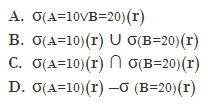# GATE | GATE CS 1999 | Question 19

The relational algebra expression equivalent to the following tuple calculus expression

### { t | t ∈ r ∧ (t[A] = 10 ∧ t[B] = 20 }

is(A) a
(B) b
(C) c
(D) d

Explanation: In Given relational algebra, Tuple t should have two attributes A=10 and B=20
In A, we select tuples having A=10 or B=20 so, it is wrong choice.
In B, we select tuples having A=10 or we can select tuples having B=20, so it is also wrong choice.
D is also wrong choice.
In C, we select tuples having A=10 and tuples having B=20, it is same as above relational algebra. Hence, option (C) is Correct.

Quiz of this Question

My Personal Notes arrow_drop_up
Article Tags :

Be the First to upvote.

Please write to us at contribute@geeksforgeeks.org to report any issue with the above content.